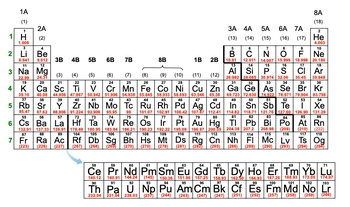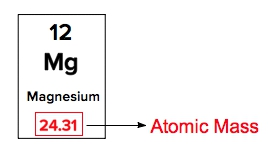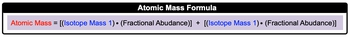## General Chemistry

Learn the toughest concepts covered in Chemistry with step-by-step video tutorials and practice problems by world-class tutors

2. Atoms & Elements

# Atomic Mass

The atomic mass of an element includes the masses of all 3 subatomic particles of neutrons, protons and electrons.

Determining Atomic Mass
1
concept

## Atomic Mass2m
Play a video:
so atomic mass is the mass of an element that includes the masses of all three subatomic particles. So we're looking at all the protons, neutrons and electrons forgiven element. We can also say that atomic masses of elements can be found by simply looking at the periodic table. So if we take a look here, the first element of the periodic table is H H stands for hydrogen. Now, later on, we'll go into how elements symbols are related to names, but for now realize that is hydrogen, this number below it. This 1.8 here that we have that is its atomic mass. And if we look, we can see that a majority of the elements have atomic masses that are not whole numbers. Well, that's because the atomic mass oven element is an average of all of its isotopes. So hydrogen has several different isotopes, and from those isotopes were taking the average mass. That's why we do not have whole numbers. Now. We can say here that these have this atomic mass can come in different units. Now we're used to one of them, and that would be grams per mole. That's our normal units for atomic mass, but can also be expressed as atomic mass units or Dalton's now recall that an atomic mass units itself is equal to 1.66 times 10 to the negative kg. So just realize when it comes to the periodic table, we have the elements symbol for each of these elements, and this number on the bottom, which is not a whole number, is the atomic mass, the number on the top, which will always be a whole number that is our atomic number. And remember, your Tomic number uses the variable Z. Okay, so keep this in mind when looking at any periodic table. Now that we've talked about the basic parts of a typical periodic table, let's continue on with additional videos.2
example

## Atomic Mass Example 12m
Play a video:
so here for this example question. It says Which of the following represents an element from the first column with the greatest atomic mass? All right, so our first column. If we look at this periodic table, our first column is this with all of these different elements. And remember the number of red, which is not a whole number. Normally, that represents the atomic mass of any of these given elements. Now here, if we take a look, we have barium. Be a again. Later, we'll learn about how the names are attached to the elements. Symbol B. A is not in the first column here. It's in the second call, so this cannot be a choice. Then we're gonna say next that we have a l A. L stands for aluminum. Aluminum is over here in the third column. Well, all the way over here in this 13th column, actually, So this is out. Next, we have C s just session. Here it is, right here. It's in the first column. It's pretty low down there. It's 1 32.91 for its atomic mass. Remember, that could be in grams per mole. Atomic mass units or Dalton's. So far, it looks like it's the highest one. The only one higher than that would be F R. Notice that in the bottom rose here. Most of them are whole numbers. These are super large mass elements that are pretty unstable. They typically don't have numerous isotopes. As a result, they have no decimal places. So next, so so far. See, looks like it's our best choice. If we look at D, we have alli, which is up here not hiring mass, not greater atomic mass. And then we have a which is right here. So it looks like, see is our best choice. It has the greatest mass atomic mass from column one from the choices provided. So just remember, we have our element symbols. We have our atomic masses, which normally are not whole numbers, and then we actually have whole numbers. Those represent our atomic numbers

On the Periodic Table, the atomic mass is represented by the number with decimal places.3
Problem

Which of the following choices has the greatest atomic mass?

4
concept

## Atomic Mass2m
Play a video:
now we can look up. The atomic mass is off a known element by simply looking at the periodic table. But there's going to be instances where you may not know the identity of the element, or you may not have a periodic table handy. In these cases, we can actually calculate the atomic mass for that element. Now, toe the atomic mass of an element can be calculated. If you know the isotopic masses and percent abundances off that element, we're going to say here that you're isotopic masses air just the masses of for all the isotopes of a given element, the percent abundances air. Just the percentage is available for each of the isotopes of a given element. Sometimes these percent abundances are also called natural abundances. Yeah, now associated with our percent or natural abundances, we have our fractional abundances. It represents the percent abundance of an isotope divided by 100. So remember when you have a percentage forms such as 25% to get its fractional form, you were divided by 100. That's what's going on here. We have our percent abundances. We can divide them by 100 that will give us our fractional abundances With this piece of information, we can use the atomic mass formula. Now. The atomic mass formula says that your atomic mass equals the isotope mass one. So the mass of your first isotope times it's fractional abundance plus isotope mass. Two times it's fractional abundances now realize that some elements have mawr than two isotopes, so you just continue on with the formula. So you say, plus isotope mass three times. It's fractional abundance and so on and so forth. So just realize that we're gonna utilize this atomic mass formula in order to calculate the atomic mass of an element when we don't have a periodic table handy.5
example

## Atomic Mass Example 22m
Play a video:
in the following example. Question, it says calculate the atomic mass of gallium if galleon has two naturally occurring isotopes with the following masses and natural abundances. So here we're dealing with gallium 69 71. Their atomic masses are written in terms of atomic mass units, and then here we have 60.11% and 39.89%. Those represent the percent abundance is now to find the atomic mass of our gallium element. Let's follow step one. Step one says, if you are given percent abundances, which we were, we're going to divide them by 100 in order to isolate our fractional abundances. So divide them both y 100. When we do that, we're gonna get our fractional abundances. So then we have these now as our fractional abundances step to plug your given variables into the atomic mass formula in order to isolate the missing variable. So this is just a simple plug and chug algebraic type of situation. Our atomic mass of our element equals the mass of the first isotope times. It's fractional abundance, plus the mass of our second isotope times. It fractional abundance. So when we do that, we're gonna get 69. am you. Since our isotopic masses have four decimal places, we could follow four decimal places here. But we have multiple choice options and we're gonna go with the best answer, which would be option A for this particular question. So using the atomic mass formula is pretty simple. All we have to do is round up all the variables that you're given and isolate the one that's missing. From there, you can find your final answer.
6
concept

## Atomic Mass40s
Play a video:
Now, how do we calculate atomic mass when no fractional abundances are given? Well, remember, adding up the fractional abundances off all isotopes for an element gives a total of one. Because remember, all of them together constitute 100% for that particular element. And dividing it by ah 100 gives us it's fractional abundance in that equals one. Now, this is important for atomic mass questions where there are two isotopes with unknown fractional abundances. So basically, if you're gonna have a question where you have to calculate the fraction abundances of all isotopes, it will only be for two isotopes, not three or more.
7
example

## Atomic Mass Example 33m
Play a video:
here. It tells us that Born has two naturally occurring isotopes Boron 10, which has a mass of 10.13 atomic mass units, and boron 11, which has a mass of 11.9 atomic mass units. He we're told, what is the natural or percent abundance off the boron 10 isotope? All right, so Step one, we make the first isotopes fractional abundance equal to one. But remember what we said earlier? The total fractional abundance of all the isotopes adds upto one right. So if you know one of them, then the second isotope one would be one minus X. And this is what you must always do. The first isotope is X. The second one is one minus X, then all we do is we plug your given variables into the atomic mass formula in order to isolate the missing variable. All right, so we're gonna plug in average mass with the atomic mass equals the mass of isotope. One times it's fractional abundance, plus the mass of isotope to times it's fractional abundance. So then we're gonna plug in the average mass or atomic mass comes from the periodic table and for bore on its 10.81. The mass of the first isotope is 10.13 atomic mass units. It's fractional abundance, we said his ex plus the mass of the second isotope, which is 11.9 times one minus X distribute, distribute, distribute. So we're gonna have 10.81 equals 10. x plus 11. minus 11.9 x. Since both of these numbers have the X variable attached to them, you're going to combine them together. So when we combine them together, we're gonna get negative. 0.996 x plus 11.9 still equals 10.81. Remember, we're trying to isolate X, so subtract out 11.9 from both sides here. When we do that, we're going to get negative. 0.199 equals negative 0.996 X isolate our extra divide both sides by negative 0.996 negative 0.996 And when we do that, we're gonna get our X for our isotope. X here equals 0.1998 which remember is your fractional abundance. We want the percent or natural abundance, so multiply it by 100. So that gives me 19.98% which is the percent abundance of my first isotope since it uses the X variable. So for Boron 10, it's natural abundances 19.98%. If you wanted to calculate the natural abundance of the second isotope, you would just say Ah 100 minus that 19. which would be 80.2%. I know I don't ask for it, but I'm just showing you how you would get the other isotope. But for this particular question, the percent abundance of boron 10 would be 19.98%.
8
Problem

Only three isotopes of magnesium exist on earth. 24Mg is the most common form at 78.70% natural abundance with a mass of 23.98504 amu, 25Mg has a 10.13% natural abundance, while 26Mg has a natural abundance of 11.17% and a mass of 25.98259 amu. What is the mass of the 25Mg isotope?

9
Problem

Silver has an atomic mass of 107.868 amu. The Ag-109 isotope (108.905 amu) is 48.16%. What is the amu of the other isotope?